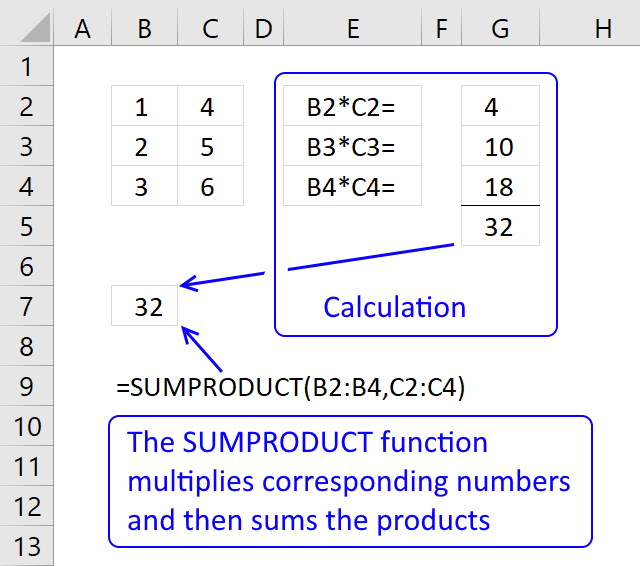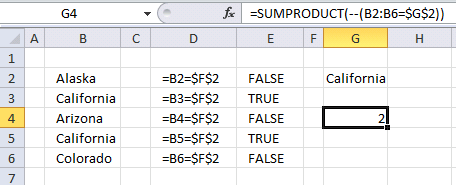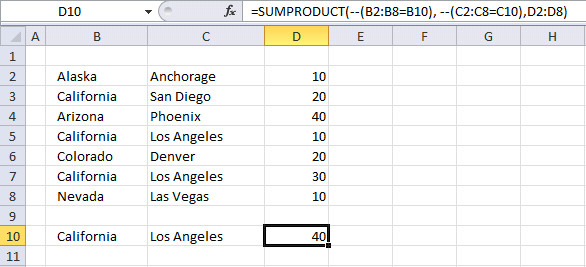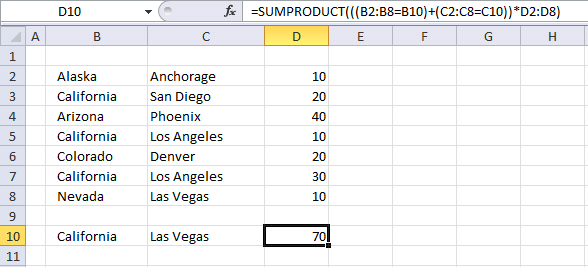Author: Oscar Cronquist Article last updated on June 16, 2018The SUMPRODUCT function calculates the product of corresponding values and then returns the sum of each multiplication.

The picture above shows how the SUMPRODUCT works in greater detail.

Excel Function Syntax

SUMPRODUCT(array1, [array2], ...)

Arguments

 array1 Required. Required. The first array argument whose numbers you want to multiply and then sum. [array2] Required. Up to 254 additional arguments.

Example 1 - The basicsFormula in cell B7:

=SUMPRODUCT(B2:B4, C2:C4)

Step 1  - Multiplying corresponding components in the given arrays

The first array is in cell range B2:B4 and the second array is in cell range C2:C4.

B2:B4*C2:C4

becomes

{1;2;3} * {4;5;6}

becomes

{1*4; 2*5; 3*6}

and returns {4; 10; 18}. The same calculations are done in column D and shown in column E, see above picture.

Step 2 - Return the sum of those products

{4; 10; 18}

becomes

4 + 10 + 18

and returns 32 in cell B7. The same calculation is done E5, the sum of the products in cell range E2:E4 is calculated in cell E5. See above picture.

Now you know the basics. Let´s move on to something more interesting!

Example 2 - Use a logical expressionFormula in cell G4:

=SUMPRODUCT(--(B2:B6=\$G\$2))

Step 1  - Multiplying corresponding components in the given arrays

There is only one array in this formula but something else is distorting the picture. A comparison operator (equal sign) and a second cell value (G2) or a comparison value. With these we have now built a logical expression. This means that the value in cell G2 is compared to all the values in cell range B2:B6 (not case sensitive).

B2:B6=\$G\$2

becomes

and returns

{FALSE;TRUE;FALSE;TRUE;FALSE}.

They are all boolean values and excel can´t sum these values. We have to convert the values to numerical values. There are a few options, you can:

• Add a zero - (B2:B6=\$G\$2)+0
• Multiply with 1 - (B2:B6=\$G\$2)*1
• Double negative signs  --(B2:B6=\$G\$2)

They all convert boolean values to numerical values.

--( {FALSE;TRUE;FALSE;TRUE;FALSE})

becomes

{0;1;0;1;0}

Step 2 - Return the sum of those products

{0;1;0;1;0}

becomes

0 + 1 + 0 + 1 + 0

and returns 2 in cell G4. There are two cells containing the value "California" in cell range B2:B6. You accomplish the same thing using the countif function or count multiple values in different columns using the countifs function. In fact, you can count entire records in a table.

Example 3 - Use multiple logical expressionsFormula in cell D10:

=SUMPRODUCT(--(B2:B8=B10), --(C2:C8=C10),D2:D8)

This formula contains three arrays, you can use up to 30 arrays. You can make the formula somewhat shorter:

=SUMPRODUCT((B2:B8=B10)*(C2:C8=C10)*D2:D8)

This allows you to have a lot more expressions, if you like. It is only the available computer memory that is the limit. The formula looks like an array formula but don´t be fooled, you are not required to enter it as an array formula.

Step 1  - Multiplying corresponding components in the given arrays

(B2:B8=B10)*(C2:C8=C10)*D2:D8

becomes

({FALSE; TRUE; FALSE; TRUE; FALSE; TRUE; FALSE})*({FALSE; FALSE; FALSE; TRUE; FALSE; TRUE; FALSE})*{10; 20; 40; 10; 20; 30; 10}

becomes

{0;0;0;1;0;1;0}*{10; 20; 40; 10; 20; 30; 10}

and returns

{0; 0; 0; 10; 0; 30; 0}

Step 2 - Return the sum of those products

{0; 0; 0; 10; 0; 30; 0}

becomes

10 +30

and returns 40 in cell D10.

Example 4 - Use multiple logical expressions and mathematical operators

Mathematical operators between arrays allows you to do more complicated calculations, like this:Formula in cell D10:

=SUMPRODUCT(((B2:B8=B10)+(C2:C8=C10))*D2:D8)

These expressions check if California is found in cell range B2:B8 or Las Vegas is found in cell range C2:C8. They are found in row 3, 5,7 and 8. The sumproduct function sums the corresponding values in column D and returns 70 in cell D10. 20+10+30+10 equals 70.